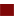STUDY
AND
EXAM
.COM

# Tips for IELTS Writing Test

In the writing part of IELTS, there are 2 tasks for writing. Time allowed for this module is 60 minutes.

Task 1 : To summarize or describe a given table, chart, graph or a diagram.
Task 2 : To write an essay on a given topic.## Tips for IELTS Writing test

The tips to score high in writing test are as follows:

1. IELTS writing test requires literary and scholarly writing skills.
2. You must be able to write sentence having grammatical accuracy.
3. Use a wide range of vocabulary. Avoid using common words. Use formal words instead of informal words.
4. You should learn the correct structure of a paragraph. The starting line of a paragraph is introductory line which introduces an idea. The next lines elaborate the idea of first line. Use one paragraph for discussing one idea. Don’t mix many ideas in a same paragraph.
5. Write short sentences. Avoid writing long sentence. Short sentences have less chances of grammatical errors.
6. The starting letter of each sentence must be capital. Each sentence should be ended with period (.). Have proper space between two sentences.
7. Be careful about correct punctuation, i.e. comma, question mark, semi-colon, exclamation mark etc.
8. To describe a table or a graph, focus on its base and vertical information. In graphs and table, there are two variable information having a relationship between them. Introduce the table in a few lines and describe the variables with regard to their inter-relationship shown in the table or graph.
9. To describe a diagram, focus on all its constituents. Diagram represents a process involving many steps. Understand the process and its step as shown in the diagram. Introduce the process and describe each of its steps as shown in diagram.
10. To write an essay on the given topic, read the topic carefully. Think about its various aspect to draw a sketch of it in mind. You can make an outline of the essay on a rough sheet to help yourself in writing the whole essay.
11. Essay has a proper structure having three parts, 1. Introduction, 2. Body, 3. Conclusion. In the first paragraph introduce the topic writing a background for the topic. The next part, the body, consists of paragraphs elaborating various aspect of the topic. In the last part, the conclusion part, summarise you whole essay in a comprehensive manner.
12. Practice! Writing part requires proper preparation before appearing the test. Hence, practice the available tests. Learn the basics of grammar. Learn good vocabulary as well as its correct usage.